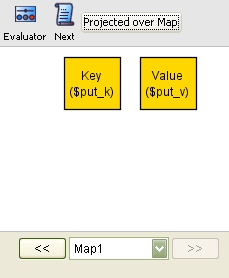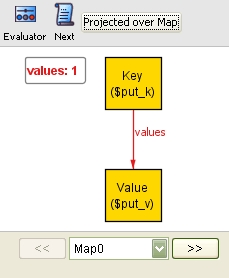A Guide to Alloy
 Home Introduction Tutorial Getting Started Statics I Statics II Dynamics I Dynamics II Alternatives to Alloy Test Yourself! Glossary FAQs Final Thoughts
Dynamics II
We will proceed to adding dynamic behaviour to our model. In particular, we will define the operation of adding entries to the Map:
`	pred put(m, m':Map, k:Key, v:Value) { m'.values = m.values + k -> v }`
This is very similar to an operation schema in Object-Z: the instances of put consist of the state before(m), inputs(v), state after(m') and outputs of the operation. As in Object-Z, the convention is to use ' to decorate the state after the operation.

+ is the set union operator. The above constraint therefore reads as:
`		"The set of values after is equal to the union of the set of values before with the extra mapping from k to v"`
(Note how m.values is again treated as a set of tuples).

We execute this predicate with the usual command:
```	run put for 3 but exactly 2 Map, exactly 1 Key, exactly 1 Value
```
Alloy produces the following instance which illustrates the functionality of the put operation:
 ```module examples/tutorial/Map abstract sig Object {} sig Key, Value extends Object {} sig Map { values: Key -> lone Value } assert mappingIsUnique { all m:Map, k:Key, v, v':Value | k -> v in m.values and k -> v' in m.values implies v = v' } pred put(m, m':Map, k:Key, v:Value) { m'.values = m.values + k -> v } run put for 3 but exactly 2 Map, exactly 1 Key, exactly 1 Value ```State beforeState after
The assertion putLocal checks that put does not change the existing mappings in the set:
```	assert putLocal {
all m, m': Map, k, k': Key, v: Value |
put[m,m',k,v] and k != k' implies
lookup[m,k'] = lookup[m',k']
}
fun lookup(m: Map, k: Key): set Value { k.(m.values) }
```
lookup is an example of a function in Alloy. It uses the . operator to return all values that are associated with the Key k in the Map m, i.e. the set {v | (k, v) in m.values}. Note that the arguments to a function are enclosed in square brackets []. This is new in Alloy 4; in older versions (such as the one used in the official tutorial on the MIT website), they were enclosed in parentheses ().

We check that the assertion holds as before:
```	check putLocal
```
Alloy informs us that no counter-examples were found. This is also the case if we increase the scope (to 15, say). Confident that our assertion may be valid, we stop here.

This already marks the end of our short tutorial. If you want to learn more about Alloy, we suggest looking at the following excellent resources: